[ Graphics Archive | Up | Comments ]

### Special Topics:Hyperbolic Geometry

The Greek geometer Euclid studied the geometry of the plane, and stated 5 axioms that he took as assumptions about the plane (for example, all right angle are equal). These were supposed to the "obvious", but he was unsatisfied with one. This axiom became known as the "parallel" postulate because it states that given a line and a point not on that line, there is exactly one line through the point parallel to the given line. This axiom was more complicated than the others, and mathematicians tried for centuries to prove if from the other four. In the nineteenth century, however, two new geometries were discovered that satisfy the first four axioms but not the fifth. In one of these, there are no parallel lines (this is the geometry of the sphere) and it is called elliptical geometry. In the other, there are many parallel lines through the given point, and this is called hyperbolic geometry. It has several representations within the unit circle, or in the upper half-plane of 2-dimensional space. There are also higher-dimensional analogs, just as there are higher-dimensional Euclidean spaces.

Subgroups:

Images:

1. 237 Tiling of the Hyperbolic Plane
2. 4 Dodecahedra in Hyperbolic Space
3. Borromean Ring Complement Manifold 1
4. Borromean Ring Complement Manifold 2
1.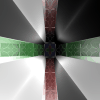2.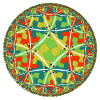3.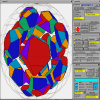4.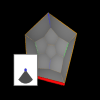1. Borromean Ring Complement Manifold 3
2. Escher Fish
3. Hyperbolic Dirichlet Domains
4. Hyperbolic Dodecahedron
1.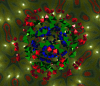2.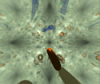3.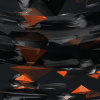4.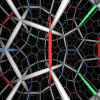1. Hyperbolic Kaleidoscope: 2-3-5
2. Hyperbolic Kaleidoscope: 3-4-4
3. Hyperbolic Kaleidoscope: S2
4. Hyperbolic Space Tiled with Dodecahedra, 1
1.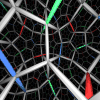2.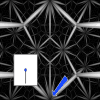3.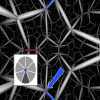1. Hyperbolic Space Tiled with Dodecahedra, 2
2. The Borromean Ring Complement Manifold
3. The Order-7 Borromean Ring OrbifoldThe Geometry Center Home Page